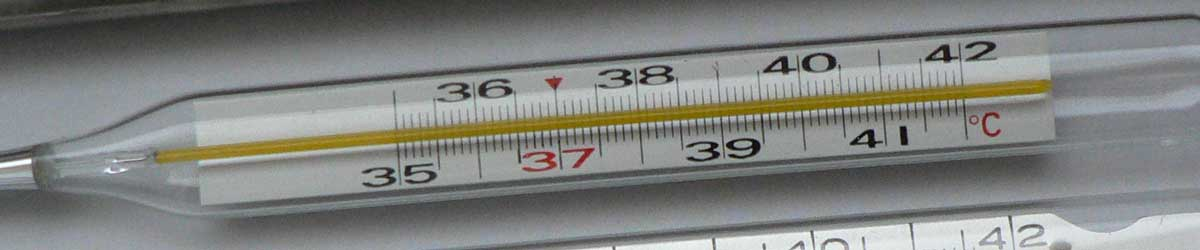# Newton to Rankine Conversion

Enter the temperature in Newton below to get the value converted to Rankine.

Results in Degrees Rankine:1 °N = 497.124545 °R
Do you want to convert Rankine to Newton?

## How to Convert Newton to RankineConvert degrees Newton to degrees Rankine with this simple formula:

degrees Rankine = [°N] × 60 / 11 + 491.67

Insert the [°N] temperature measurement in the formula and then solve to find the result.

For example, let's convert 50 °N to degrees Rankine:

50 °N = ( 50 × 60 / 11 + 491.67 ) = 764.397273 °R

## Newton

The Newton scale is a temperature scale that sets a fixed zero point equal to the freezing point water. The scale uses both arithmetic progression and geometric progression. The scale defines 18 reference points and the corresponding "degrees of heat" and geometric progressions.

Newton can be abbreviated as N; for example, 1 degree Newton can be written as 1 °N.

## Rankine

The Rankine scale is an absolute temperature scale that uses absolute zero as the starting point, much like the Kelvin scale. The key difference between the Rankine scale and the Kelvin scale is that an increment of one degree rankine is equal to an increment of one degree Fahrenheit, not Celsius as in the Kelvin scale. The base of the Rankine scale starts at absolute zero, thus 0 degrees rankine are equal to −459.67 degrees Fahrenheit.

Rankine can be abbreviated as R, and are also sometimes abbreviated as Ra. For example, 1 degree Rankine can be written as 1 °R or 1 Ra.

### Important Temperatures In Degrees Rankine

TemperatureDegrees Rankine
Absolute Zero0 °R
Freezing Point of Water491.67 °R
Triple Point of Water491.688 °R
Boiling Point of Water671.67 °R

## Newton to Rankine Conversion Table

Newton measurements converted to Rankine
Newton Rankine
-90.14 °N 0 °R
-50 °N 218.94 °R
-40 °N 273.49 °R
-30 °N 328.03 °R
-20 °N 382.58 °R
-10 °N 437.12 °R
0 °N 491.67 °R
10 °N 546.22 °R
20 °N 600.76 °R
30 °N 655.31 °R
40 °N 709.85 °R
50 °N 764.4 °R
60 °N 818.94 °R
70 °N 873.49 °R
80 °N 928.03 °R
90 °N 982.58 °R
100 °N 1,037 °R
110 °N 1,092 °R
120 °N 1,146 °R
130 °N 1,201 °R
140 °N 1,255 °R
150 °N 1,310 °R
160 °N 1,364 °R
170 °N 1,419 °R
180 °N 1,473 °R
190 °N 1,528 °R
200 °N 1,583 °R
300 °N 2,128 °R
400 °N 2,673 °R
500 °N 3,219 °R
600 °N 3,764 °R
700 °N 4,310 °R
800 °N 4,855 °R
900 °N 5,401 °R
1,000 °N 5,946 °R